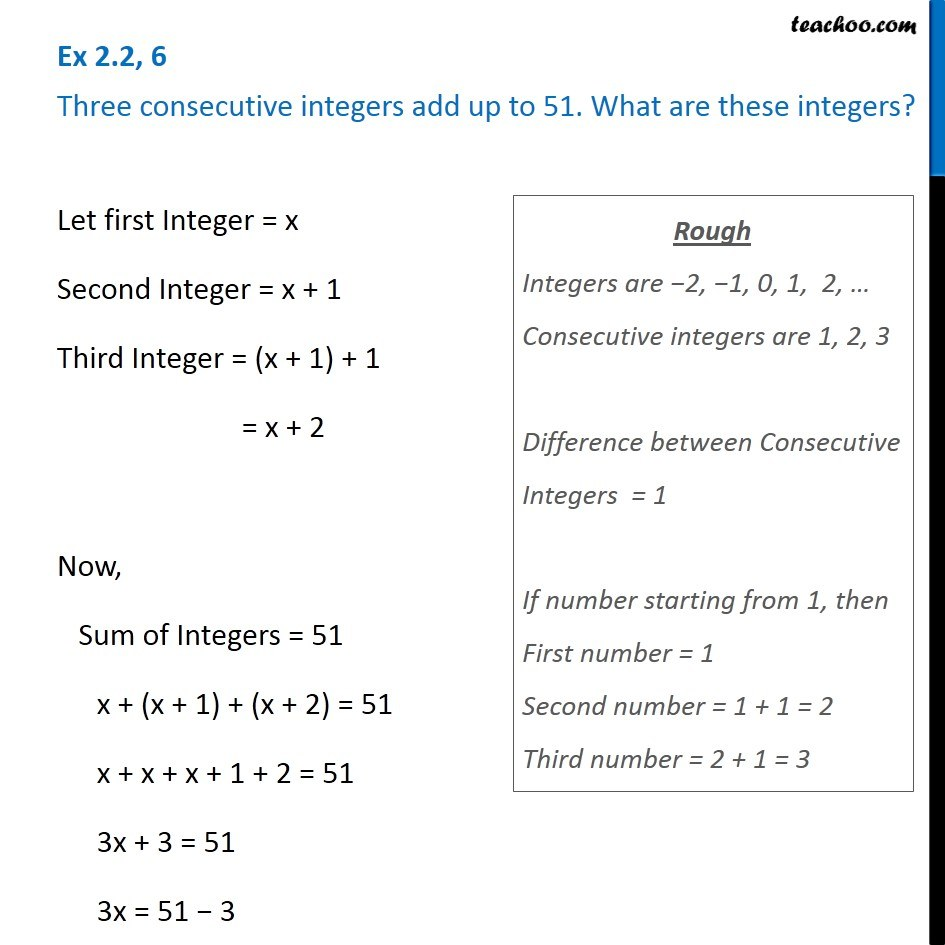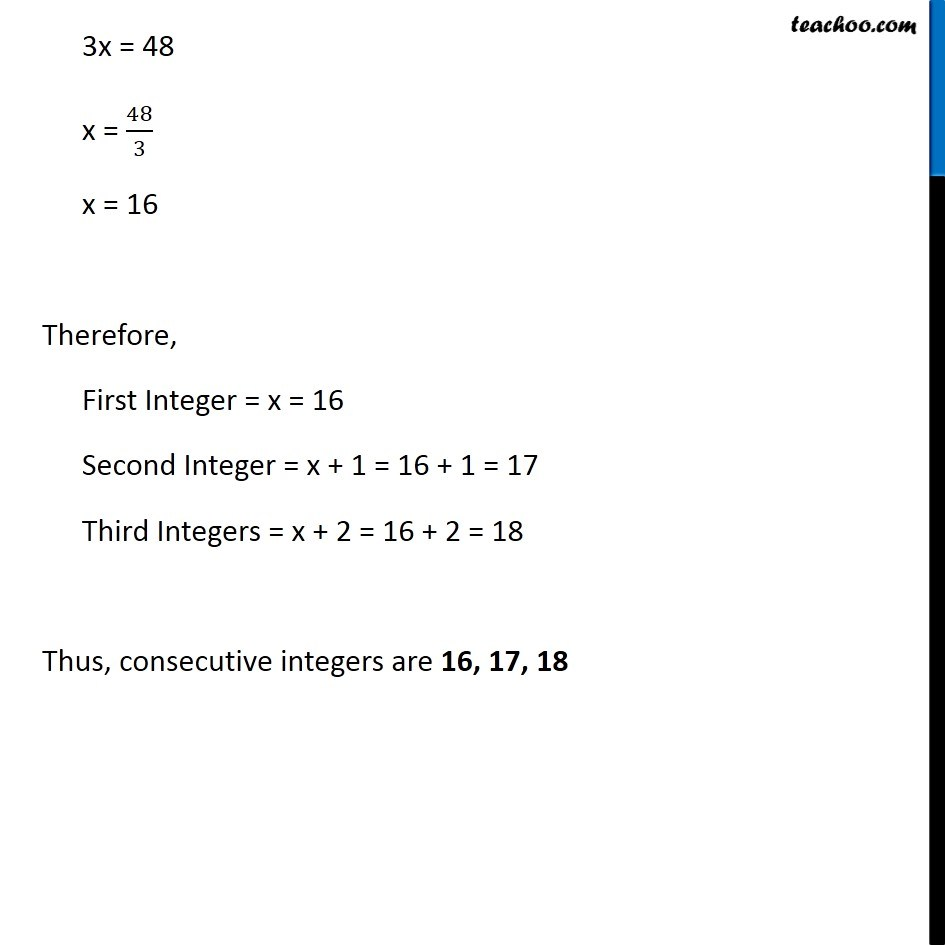Solving easy equations - Word Problems

Chapter 2 Class 8 Linear Equations in One Variable
Serial order wiseLearn in your speed, with individual attention - Teachoo Maths 1-on-1 Class

### Transcript

Question 6 Three consecutive integers add up to 51. What are these integers?Let first Integer = x Second Integer = x + 1 Third Integer = (x + 1) + 1 = x + 2 Now, Sum of Integers = 51 x + (x + 1) + (x + 2) = 51 x + x + x + 1 + 2 = 51 3x + 3 = 51 3x = 51 − 3 Rough Integers are −2, −1, 0, 1, 2, … Consecutive integers are 1, 2, 3 Difference between Consecutive Integers = 1 If number starting from 1, then First number = 1 Second number = 1 + 1 = 2 Third number = 2 + 1 = 3 3x = 48 x = 48/3 x = 16 Therefore, First Integer = x = 16 Second Integer = x + 1 = 16 + 1 = 17 Third Integers = x + 2 = 16 + 2 = 18 Thus, consecutive integers are 16, 17, 18